• 本篇内容就是关于回归模型的评价，首先介绍线性回归模型的三个常用评价方法，然后通过波士顿房产预测的实际例子，对评价方法进行代码实现。最后我们会隆重引出最好的衡量线性回归法的指标：R Square 1.线性回归算法...
本篇内容就是关于回归模型的评价，首先介绍线性回归模型的三个常用评价方法，然后通过波士顿房产预测的实际例子，对评价方法进行代码实现。最后我们会隆重引出最好的衡量线性回归法的指标：R Square
1.线性回归算法的衡量标准
简单线性回归的目标是：已知训练数据样本x、y ，找到a和b的值，使Σ(y-ax-b)²  尽可能小
衡量标准是看在测试数据集中y的真实值与预测值之间的差距。
但是这里有一个问题，这个衡量标准是和数据量m相关的。在具体衡量时，测试数据集不同将会导致误差的累积量不同。
在得到a和b之后将x(test)代入a、b中。可以使用Σ(y(test)-ax(test)-b)²来作为衡量回归算法好坏的标准。
1.1均方误差MSE
测试集中的数据量m不同，因为有累加操作，所以随着数据的增加 ，误差会逐渐积累；因此衡量标准和 m 相关。为了抵消掉数据量的形象，可以除去数据量，抵消误差。通过这种处理方式得到的结果叫做 均方误差MSE（Mean Squared Error）
1.2 均方根误差RMSE
但是使用均方误差MSE收到量纲的影响。例如在衡量房产时，y的单位是（万元），那么衡量标准得到的结果是（万元平方）。为了解决量纲的问题，可以将其开方（为了解决方差的量纲问题，将其开方得到平方差）得到均方根误差RMSE（Root Mean Squarde Error）
1.3 平均绝对误差MAE
对于线性回归算法还有另外一种非常朴素评测标准。要求真实值  与 预测结果  之间的距离最小，可以直接相减做绝对值，加m次再除以m，即可求出平均距离，被称作平均绝对误差MAE（Mean Absolute Error）
确定损失函数时，绝对值函数不是处处可导的，因此没有使用绝对值。但是在评价模型时不影响。因此模型的评价方法可以和损失函数不同。
2.评价标准的代码实现
2.1 数据探索
import numpy as np
import matplotlib.pyplot as plt
from sklearn import datasets

# 查看数据集描述
boston = datasets.load_boston()
print(boston.DESCR)
.. _boston_dataset:

Boston house prices dataset
---------------------------

**Data Set Characteristics:**

:Number of Instances: 506

:Number of Attributes: 13 numeric/categorical predictive. Median Value (attribute 14) is usually the target.

:Attribute Information (in order):
- CRIM     per capita crime rate by town
- ZN       proportion of residential land zoned for lots over 25,000 sq.ft.
- INDUS    proportion of non-retail business acres per town
- CHAS     Charles River dummy variable (= 1 if tract bounds river; 0 otherwise)
- NOX      nitric oxides concentration (parts per 10 million)
- RM       average number of rooms per dwelling
- AGE      proportion of owner-occupied units built prior to 1940
- DIS      weighted distances to five Boston employment centres
- RAD      index of accessibility to radial highways
- TAX      full-value property-tax rate per $10,000 - PTRATIO pupil-teacher ratio by town - B 1000(Bk - 0.63)^2 where Bk is the proportion of blacks by town - LSTAT % lower status of the population - MEDV Median value of owner-occupied homes in$1000's

:Missing Attribute Values: None

:Creator: Harrison, D. and Rubinfeld, D.L.

This is a copy of UCI ML housing dataset.
https://archive.ics.uci.edu/ml/machine-learning-databases/housing/

This dataset was taken from the StatLib library which is maintained at Carnegie Mellon University.

The Boston house-price data of Harrison, D. and Rubinfeld, D.L. 'Hedonic
prices and the demand for clean air', J. Environ. Economics & Management,
vol.5, 81-102, 1978.   Used in Belsley, Kuh & Welsch, 'Regression diagnostics
...', Wiley, 1980.   N.B. Various transformations are used in the table on
pages 244-261 of the latter.

The Boston house-price data has been used in many machine learning papers that address regression
problems.

.. topic:: References

- Belsley, Kuh & Welsch, 'Regression diagnostics: Identifying Influential Data and Sources of Collinearity', Wiley, 1980. 244-261.
- Quinlan,R. (1993). Combining Instance-Based and Model-Based Learning. In Proceedings on the Tenth International Conference of Machine Learning, 236-243, University of Massachusetts, Amherst. Morgan Kaufmann.

因为是测试简单回归算法，因此我们选择其中的一个特征进行建模。选择：

RM average number of rooms per dwelling 每个住宅的平均房间数
下面我们进行简单的数据探索：

# 查看数据集的特征列表
boston.feature_names
array(['CRIM', 'ZN', 'INDUS', 'CHAS', 'NOX', 'RM', 'AGE', 'DIS', 'RAD',
'TAX', 'PTRATIO', 'B', 'LSTAT'], dtype='<U7')

# 取出数据中的第六例的所有行（房间数量）
x = boston.data[:,5]
x.shape
(506,)

# 取出样本标签
y = boston.target
y.shape
(506,)

plt.scatter(x,y)
plt.show()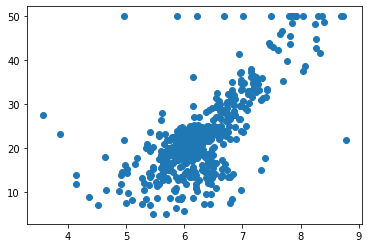在图中可以看到 50W 美元的档分布着一些点。这些点可能是超出了限定范围（比如在问卷调查中，价格的最高档位是“50万及以上”，那么就全都划到50W上了，因此在本例中，可以将这部分数据去除）
np.max(y)
# 这里有一个骚操作，用比较运算符返回一个布尔值的向量，将其作为索引，直接在矩阵里对每个元素进行过滤。
x = x[y < 50.0]
y = y[y < 50.0]
plt.scatter(x,y)
plt.show()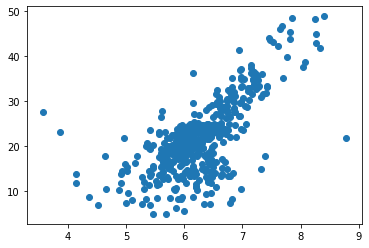2.2 简单线性回归预测
from model_selection import train_test_split

x_train, x_test, y_train, y_test = train_test_split(x, y, seed=666)
print(x_train.shape)    # (392,)
print(y_train.shape)    #(392,)
print(x_test.shape)     #(98,)
print(y_test.shape)     #(98,)
(392,)
(392,)
(98,)
(98,)

from SimpleLinearRegression import SimpleLinearRegression

reg = SimpleLinearRegression()
reg.fit(x_train,y_train)
print(reg.a_)   # 7.8608543562689555
print(reg.b_)   # -27.459342806705543
7.860854356268954
-27.459342806705536

plt.scatter(x_train,y_train)
plt.plot(x_train, reg.predict(x_train),color='r')
plt.show()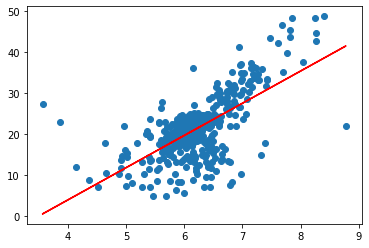y_predict = reg.predict(x_test)
print(y_predict)
[23.09381156 23.14883754 19.20268865 29.02089574 25.6014241   5.06887252
24.66598243 26.47397893 15.52380881 28.38416654 17.29250104 13.0633614
23.99780981 21.37228445 23.29033292 21.66313607 21.22292822 19.94946982
22.41777808 25.17693796 19.51712283 24.14716604 24.57165218 19.07691498
23.14097668 28.78507011 20.46042535 18.18863844 15.93257324 29.46110359
31.65428195 19.36776659 16.77368466 38.07659996 19.72936589 20.99496345
18.27510784 24.22577459 21.67099692 22.7086297  21.22292822 19.17910609
15.41375685 19.41493172 16.5771633  23.13311583 23.71481905 30.13713706
17.99211708 24.69742585 19.43065343 25.4284853  22.71649055 16.53785903
19.13194096 18.82536764 22.15836989 18.55809859 25.03544258 29.52399042
18.75461995 19.69006162 18.59740287 14.58836714 18.81750679 24.65812158
20.83774636 19.77653102 27.88893272 19.32846232 22.9837596  22.86584678
25.3262942  22.19767416 26.25387501 24.6188173  20.45256449 16.55358073
14.2346287  26.19884903 35.96989099 19.62717479 21.01854601 15.42947856
20.90849405 20.24818228 21.79677059 27.37797718 22.65360371 18.69959397
23.5340194  27.31509035 32.63688875 20.02807836 19.43851428 30.38082355
31.13546556 25.00399917]

2.3 MSE 均方误差
mse_test = np.sum((y_predict - y_test) ** 2) / len(y_test)
mse_test
24.156602134387438

2.4 RMSE 均方根误差
from math import sqrt

rmse_test = sqrt(mse_test)
rmse_test
4.914936635846635

RMSE消除了量纲的差异，输出的结果是4.9，与y的量纲相同。解释为在RMSE指标下，我们预测的房产数据平均误差在4.9万美元左右。
2.5 MAE 平均绝对误差
mae_test = np.sum(np.absolute(y_predict - y_test)) / len(y_test)
mae_test
3.543097440946387

在MAE指标下，我们预测的房产数据平均误差在3.54万美元左右。我们看到MAE指标得到的误差要比RMSE指标得到的误差小。说明不同的评价指标的结果不同。
从数学角度来分析，RMSE和MAE的量纲相同，但RMSE的结果较大，这是因为RMSE是将错误值平方，平方操作会放大样本中预测结果和真实结果较大的差距。MAE没有放大。而我们就是要解决目标函数最大差距，因为选RMSE更好一点。
3.封装及调用
3.1 在工程文件中封装
在工程文件的metrics.py中添加以上评价指标：
import numpy as np
from math import sqrt

def accuracy_score(y_true, y_predict):
"""计算y_true和y_predict之间的准确率"""
assert y_true.shape != y_predict.shape, \
"the size of y_true must be equal to the size of y_predict"
return sum(y_true == y_predict) / len(y_true)

def mean_squared_error(y_true, y_predict):
"""计算y_true和y_predict之间的MSE"""
assert len(y_true) == len(y_predict), \
"the size of y_true must be equal to the size of y_predict"
return np.sum((y_true - y_predict) ** 2) / len(y_true)

def root_mean_squared_error(y_true, y_predict):
"""计算y_true和y_predict之间的RMSE"""
return sqrt(mean_squared_error(y_true, y_predict))

def mean_absolute_error(y_true, y_predict):
"""计算y_true和y_predict之间的MAE"""
assert len(y_true) == len(y_predict), \
"the size of y_true must be equal to the size of y_predict"

return np.sum(np.absolute(y_predict - y_true)) / len(y_predict)
3.2 调用
from metrics import mean_squared_error
from metrics import root_mean_squared_error
from metrics import mean_absolute_error

mean_squared_error(y_test, y_predict)
24.156602134387438

root_mean_squared_error(y_test, y_predict)
4.914936635846635

mean_absolute_error(y_test, y_predict)
3.543097440946387

3.3 sklearn中的MSE和MAE
sklearn中不存在RMSE，我们可以手动对MSE开方：
from sklearn.metrics import mean_squared_error
from sklearn.metrics import mean_absolute_error

mean_squared_error(y_test, y_predict)
24.156602134387438

mean_absolute_error(y_test, y_predict)
3.543097440946387

4.更好用的 R Square
4.1 R Square介绍以及为什么好
分类准确率，就是在01之间取值。但RMSE和MAE没有这样的性质，得到的误差。因此RMSE和MAE就有这样的局限性，比如我们在预测波士顿方差，RMSE值是4.9（万美元） 我们再去预测身高，可能得到的误差是10（厘米），我们不能说后者比前者更准确，因为二者的量纲根本就不是一类东西。
其实这种局限性，可以被解决。用一个新的指标R Squared。
R方这个指标为什么好呢？

对于分子来说，预测值和真实值之差的平方和，即使用我们的模型预测产生的错误。
对于分母来说，是均值和真实值之差的平方和，即认为“预测值=样本均值”这个模型（Baseline Model）所产生的错误。
我们使用Baseline模型产生的错误较多，我们使用自己的模型错误较少。因此用1减去较少的错误除以较多的错误，实际上是衡量了我们的模型拟合住数据的地方，即没有产生错误的相应指标。

我们根据上述分析，可以得到如下结论：

R^2 <= 1
R2越大也好，越大说明减数的分子小，错误率低；当我们预测模型不犯任何错误时，R2最大值1
当我们的模型等于基准模型时，R^2 = 0
如果R^2 < 0，说明我们学习到的模型还不如基准模型。此时，很有可能我们的数据不存在任何线性关系。

4.2 R Square实现
如果分子分母同时除以m，我们会发现，分子就是之前介绍过的均方误差，分母实际上是y这组数据对应的方差
1 - mean_squared_error(y_test, y_predict) / np.var(y_test)
0.6129316803937322

下面我们在工程文件metrics.py中添加自己实现的r2_score方法：
from metrics import r2_score
r2_score(y_test, y_predict)
0.6129316803937322

其实这跟掉sklearn中的方法相同：
from sklearn.metrics import r2_score
r2_score(y_test, y_predict)
0.6129316803937324

线性回归的评价指标与分类的评价指标有很大的不同，本篇介绍了均方误差MSE（预测值与真实值之差的平方和，再除以样本量）、均方根误差RMSE（为了消除量纲，将MSE开方）、平均绝对误差MAE（预测值与真实值之差的绝对值，再除以样本量）、以及非常重要的、效果非常好的R方（因此用1减去较少的错误除以较多的错误，实际上是衡量了我们的模型拟合住数据的地方，即没有产生错误的相应指标）。
在实际应用过程中，需要这些评价指标，来判别模型的好坏。


展开全文机器学习
• 文章目录分类常用指标基于混淆矩阵的评价指标AUC回归模型常用评价指标均误差方（MSE）平均绝对误差（MAE）平均绝对比例误差（MAPE）R方 分类常用指标 基于混淆矩阵的评价指标 以二分类为例，由于模型预测存在误差，...
文章目录分类常用指标基于混淆矩阵的评价指标AUC回归模型常用评价指标均误差方（MSE）平均绝对误差（MAE）平均绝对比例误差（MAPE）R方
分类常用指标
基于混淆矩阵的评价指标
以二分类为例，由于模型预测存在误差，真实值和预测值之间存在差异，于是有以下四种情况：

真实值是positive，模型预测为positive（True Positive=TP）
真实值是positive，模型预测为negative（False Negative=FN）
真实值是negative，模型预测为positive（False Positive=FP）
真实值是negative，模型预测为negative（True Negative=TN）

将这四个指标一起呈现在表格中，得到的矩阵，称它为混淆矩阵（Confusion Matrix）：要保证一个模型的效果好，也就是希望混淆矩阵第二、四象限对应的位置的数值越多越好。
基于混淆矩阵，引出以下三种评价指标

名称
公式
意义

准确率
Accurancy = $\frac{TP+TN}{TP+TN+FP+FN}$
模型所有的正确预测结果占总数的比例

精确率
precision=$\frac{TP}{TP+FP}$
精确率是针对我们预测结果而言的,它表示的是预测为正的样本中有多少是真正的正样本。精确率低说明存在大量的假正例(FP)。

召回率
Recall=$\frac{TP}{TP+FN}$
召回率是针对我们原来的样本而言的，表示的是样本中的正例有多少被预测正确了。

基于精确率和召回率，又引入了F1 Score，在样本不平衡时，宜采用该评价指标。
$F1 \quad Score = \frac{2PR}{P+R}$
其中，P代表Precision，R代表Recall。
F1-Score指标综合了Precision与Recall的产出的结果。F1-Score的取值范围从0到1的，1代表模型的输出最好，0代表模型的输出结果最差。
举例子分析，在sklearn中可以使用classification_report生成评价指标报告：
from sklearn.metrics import classification_report
print(classification_report(pred_y,y))从上述结果看出：

正例样本的精确率为0.83，说明预测为正例的样本中只要83%是正真的正例，说明预测结果存在17%假正例（将0误分类为1）。

召回率0.97，说明训练样本数据中的正例有97%被预测对了。只有3%的正样本被预测为负类。
基于以上，我们应该思考为什么模型会将0误分类为1。

AUC
AUC（Area Under Curve），这里的Curve指的就是ROC曲线，AUC就是ROC曲线下面的面积，只适用于二分类。
在上述的混淆矩阵，引入两个概念：

True Positive Rate（TPR，真阳率），所有真实类别为1的样本中，预测类别为1的比例，计算公式如下：
$TPR = \frac{TP}{TP+FN}$

False Positive Rate（FPR, 伪阳率),所有真实类别为0的样本中，预测类别为1的比例,计算公式如下：
$FPR = \frac{FP}{TP+TN}$

接下来通过例子说明如何计算AUC。数据来源：机器学习基础：AUC
（1）对于二分类问题，对于预测结果(概率)进行从大到小排序：（2）分别以从小到大的预测概率作为阈值，进行分类，如以0.2作为阈值，则21，18号样本为负例，其余样本均为正例。取不同的阈值得到不同的混淆矩阵。得到不同的（TPR，FPR）。（3）将这些（TPR，FPR）在坐标中画出来。将这些点平滑的画出，得到ROC曲线。AUC就是ROC曲线下面的面积。
最后说说AUC的优势，AUC的计算方法同时考虑了分类器对于正例和负例的分类能力，在样本不平衡的情况下，依然能够对分类器作出合理的评价。
sklearn相关函数
import numpy as np
from sklearn import metrics
y = np.array([1, 1, 2, 2])
scores = np.array([0.1, 0.4, 0.35, 0.8])
fpr, tpr, thresholds = metrics.roc_curve(y, scores, pos_label=2)
print(fpr,'\n',tpr,'\n',thresholds)
print(metrics.auc(fpr,tpr))

在这里插入图片描述
回归模型常用评价指标
均误差方（MSE）
所有样本的样本误差的平方的均值
$MSE = \frac{1}{m} \sum(y_i -\hat y_i)^2$
sklearn 相关函数：
from sklearn.metrics import mean_squared_error

平均绝对误差（MAE）
所有样本的样本误差的绝对值的均值
$MAE = \frac{1}{m} \sum|y_i - \hat y_i|$
sklearn 相关函数：
from sklearn.metrics import mean_absolute_error

平均绝对比例误差（MAPE）
所有样本的样本误差的绝对值占实际值的比例，mape越接近0，模型越准确。
$MAPE = \frac{100 \%}{n} \sum |\frac{y_i - \hat y_i}{y_i}|$
R方
因变量的方差能被自变量解释的程度，R方越接近1，则代表自变量对因变量的解释度越高。
$R^2 = 1- \frac{MSE(y,\hat y)}{Var(y)}$
sklearn 相关函数：
from sklearn.metrics import r2_score



展开全文机器学习
• 2020-06-22 00:07:26 本文首发于知乎 ...众所周知，机器学习分类模型常用评价指标有Accuracy, Precision, Recall和F1-score，而回归模型最常用指标有MAE和RMSE。...多分类模型和二分类模型的评价指标有啥区别？...
 2020-06-22 00:07:26本文首发于知乎
https://zhuanlan.zhihu.com/p/147663370

作者 | NaNNN

编辑 | 丛末

1

前言

众所周知，机器学习分类模型常用评价指标有Accuracy, Precision, Recall和F1-score，而回归模型最常用指标有MAE和RMSE。但是我们真正了解这些评价指标的意义吗？

在具体场景（如不均衡多分类）中到底应该以哪种指标为主要参考呢？多分类模型和二分类模型的评价指标有啥区别？多分类问题中，为什么Accuracy = micro precision = micro recall = micro F1-score? 什么时候用macro, weighted, micro precision/ recall/ F1-score?

这几天为了回复严谨（划去: 刁难）的reviewer，我查阅了一些文章，总算是梳理清楚啦。在这里分享给大家，权当做个总结。今天要讲的主要分为以下两点：

二分类模型的常见指标快速回顾
多分类模型的常见指标详细解析
在探讨这些问题前，让我们先回顾一下最常见的指标Accuracy到底有哪些不足。

Accuracy是分类问题中最常用的指标，它计算了分类正确的预测数与总预测数的比值。但是，对于不平衡数据集而言，Accuracy并不是一个好指标。为啥？

假设我们有100张图片，其中91张图片是「狗」，5张是「猫」，4张是「猪」，我们希望训练一个三分类器，能正确识别图片里动物的类别。其中，狗这个类别就是大多数类 (majority class)。当大多数类中样本（狗）的数量远超过其他类别（猫、猪）时，如果采用Accuracy来评估分类器的好坏，那么即便模型性能很差 (如无论输入什么图片，都预测为「狗」)，也可以得到较高的Accuracy Score（如91%）。此时，虽然Accuracy Score很高，但是意义不大。当数据异常不平衡时，Accuracy评估方法的缺陷尤为显著。

因此，我们需要引入Precision （精准度），Recall （召回率）和F1-score评估指标。考虑到二分类和多分类模型中，评估指标的计算方法略有不同，我们将其分开讨论。

2

二分类模型的常见指标快速回顾

在二分类问题中，假设该样本一共有两种类别：Positive和Negative。当分类器预测结束，我们可以绘制出混淆矩阵（confusion matrix）。其中分类结果分为如下几种：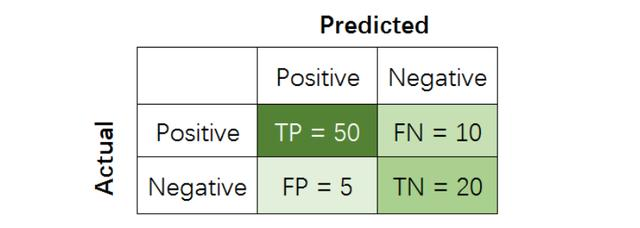True Positive (TP): 把正样本成功预测为正。
True Negative (TN)：把负样本成功预测为负。
False Positive (FP)：把负样本错误地预测为正。
False Negative (FN)：把正样本错误的预测为负。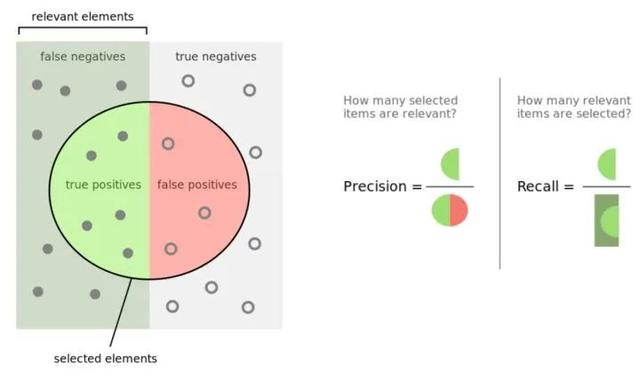在二分类模型中，Accuracy，Precision，Recall和F1 score的定义如下：

其中，Precision着重评估在预测为Positive的所有数据中，真实Positve的数据到底占多少？Recall着重评估：在所有的Positive数据中，到底有多少数据被成功预测为Positive?

举个例子，一个医院新开发了一套癌症AI诊断系统，想评估其性能好坏。我们把病人得了癌症定义为Positive，没得癌症定义为Negative。那么， 到底该用什么指标进行评估呢？

如用Precision对系统进行评估，那么其回答的问题就是：

在诊断为癌症的一堆人中，到底有多少人真得了癌症？

如用Recall对系统进行评估，那么其回答的问题就是：

在一堆得了癌症的病人中，到底有多少人能被成功检测出癌症？

如用Accuracy对系统进行评估，那么其回答的问题就是：

在一堆癌症病人和正常人中，有多少人被系统给出了正确诊断结果（患癌或没患癌）？

OK，那啥时候应该更注重Recall而不是Precision呢？

当False Negative (FN)的成本代价很高 (后果很严重)，希望尽量避免产生FN时，应该着重考虑提高Recall指标。

在上述例子里，False Negative是得了癌症的病人没有被诊断出癌症，这种情况是最应该避免的。我们宁可把健康人误诊为癌症 (FP)，也不能让真正患病的人检测不出癌症 (FN) 而耽误治疗离世。在这里，癌症诊断系统的目标是：尽可能提高Recall值，哪怕牺牲一部分Precision。

那啥时候应该更注重Precision而不是Recall呢？

当False Positive (FP)的成本代价很高 (后果很严重)时，即期望尽量避免产生FP时，应该着重考虑提高Precision指标。

以垃圾邮件屏蔽系统为例，垃圾邮件为Positive，正常邮件为Negative，False Positive是把正常邮件识别为垃圾邮件，这种情况是最应该避免的（你能容忍一封重要工作邮件直接进了垃圾箱，被不知不觉删除吗？）。我们宁可把垃圾邮件标记为正常邮件 (FN)，也不能让正常邮件直接进垃圾箱 (FP)。在这里，垃圾邮件屏蔽系统的目标是：尽可能提高Precision值，哪怕牺牲一部分recall。

而F1-score是Precision和Recall两者的综合。

举个更有意思的例子（我拍脑袋想出来的，绝对原创哈），假设检察机关想将罪犯捉拿归案，需要对所有人群进行分析，以判断某人犯了罪（Positive），还是没犯罪（Negative）。显然，检察机关希望不漏掉一个罪人（提高recall），也不错怪一个好人（提高precision），所以就需要同时权衡recall和precision两个指标。

尤其在上个世纪，中国司法体制会更偏向Recall，即「天网恢恢，疏而不漏，任何罪犯都插翅难飞」。而西方司法系统会更偏向Precision，即「绝不冤枉一个好人，但是难免有罪犯成为漏网之鱼，逍遥法外」。到底是哪种更好呢？显然，极端并不可取。Precision和Recall都应该越高越好，也就是F1应该越高越好。

呼，二分类问题的常见指标和试用场景终于讲完了。咦，说好的快速回顾呢？

3

多分类模型的常见指标解析

在多分类（大于两个类）问题中，假设我们要开发一个动物识别系统，来区分输入图片是猫，狗还是猪。给定分类器一堆动物图片，产生了如下结果混淆矩阵。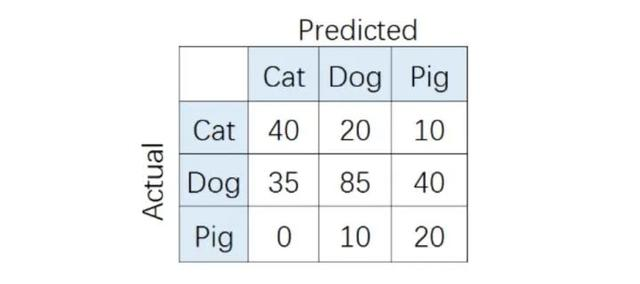在混淆矩阵中，正确的分类样本（Actual label = Predicted label）分布在左上到右下的对角线上。其中，Accuracy的定义为分类正确（对角线上）的样本数与总样本数的比值。Accuracy度量的是全局样本预测情况。而对于Precision和Recall而言，每个类都需要单独计算其Precision和Recall。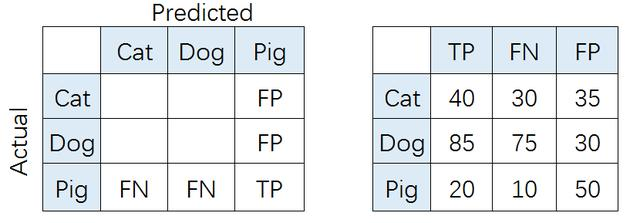比如，对类别「猪」而言，其Precision和Recall分别为:

也就是，

（P代表Precision）

（R代表Recall）

如果想评估该识别系统的总体功能，必须考虑猫、狗、猪三个类别的综合预测性能。那么，到底要怎么综合这三个类别的Precision呢？是简单加起来做平均吗？通常来说， 我们有如下几种解决方案（也可参考scikit-learn官网）：

1、Macro-average方法

该方法最简单，直接将不同类别的评估指标（Precision/ Recall/ F1-score）加起来求平均，给所有类别相同的权重。该方法能够平等看待每个类别，但是它的值会受稀有类别影响。

2、 Weighted-average方法

该方法给不同类别不同权重（权重根据该类别的真实分布比例确定），每个类别乘权重后再进行相加。该方法考虑了类别不平衡情况，它的值更容易受到常见类（majority class）的影响。

(W代表权重，N代表样本在该类别下的真实数目)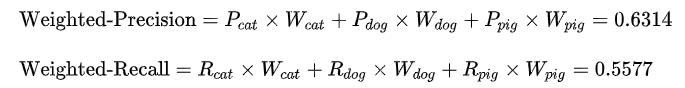3、Micro-average方法

该方法把每个类别的TP, FP, FN先相加之后，在根据二分类的公式进行计算。

其中，特别有意思的是，Micro-precision和Micro-recall竟然始终相同！这是为啥呢？

这是因为在某一类中的False Positive样本，一定是其他某类别的False Negative样本。听起来有点抽象？举个例子，比如说系统错把「狗」预测成「猫」，那么对于狗而言，其错误类型就是False Negative，对于猫而言，其错误类型就是False Positive。于此同时，Micro-precision和Micro-recall的数值都等于Accuracy，因为它们计算了对角线样本数和总样本数的比值，总结就是：

最后，我们运行一下代码，检验手动计算结果是否和Sklearn包结果一致：

import numpy as np
import seaborn as sns
from sklearn.metrics import confusion_matrix
import pandas as pd
import matplotlib.pyplot as plt
from sklearn.metrics import accuracy_score, average_precision_score,precision_score,f1_score,recall_score
# create confusion matrix
y_true = np.array([-1]*70 + *160 + *30)
y_pred = np.array([-1]*40 + *20 + *20 +
[-1]*30 + *80 + *30 +
[-1]*5 + *15 + *20)
cm = confusion_matrix(y_true, y_pred)
conf_matrix = pd.DataFrame(cm, index=['Cat','Dog','Pig'], columns=['Cat','Dog','Pig'])
# plot size setting
fig, ax = plt.subplots(figsize = (4.5,3.5))
sns.heatmap(conf_matrix, annot=True, annot_kws={"size": 19}, cmap="Blues")
plt.ylabel('True label', fontsize=18)
plt.xlabel('Predicted label', fontsize=18)
plt.xticks(fontsize=18)
plt.yticks(fontsize=18)
plt.savefig('confusion.pdf', bbox_inches='tight')
plt.show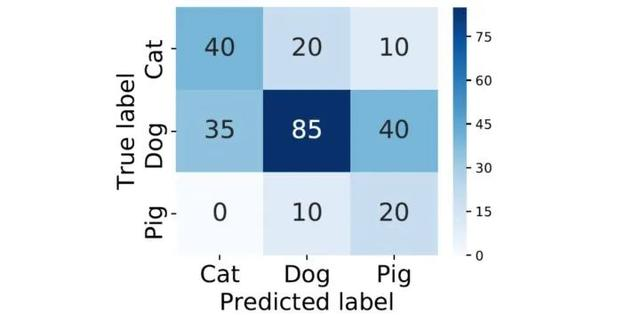print('------Weighted------')
print('Weighted precision', precision_score(y_true, y_pred, average='weighted'))
print('Weighted recall', recall_score(y_true, y_pred, average='weighted'))
print('Weighted f1-score', f1_score(y_true, y_pred, average='weighted'))
print('------Macro------')
print('Macro precision', precision_score(y_true, y_pred, average='macro'))
print('Macro recall', recall_score(y_true, y_pred, average='macro'))
print('Macro f1-score', f1_score(y_true, y_pred, average='macro'))
print('------Micro------')
print('Micro precision', precision_score(y_true, y_pred, average='micro'))
print('Micro recall', recall_score(y_true, y_pred, average='micro'))
print('Micro f1-score', f1_score(y_true, y_pred, average='micro'))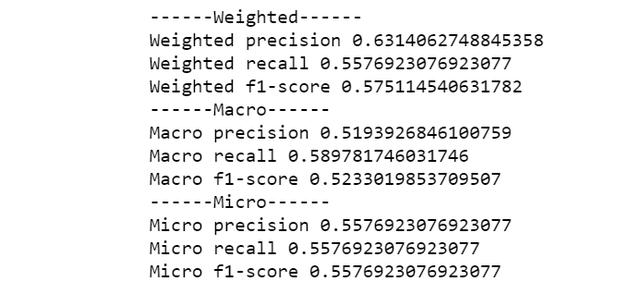运算结果完全一致，OK，机器学习多分类模型的常见评估指标已经基本介绍完毕。

参考文章

4 Things You Need to Know about AI: Accuracy, Precision, Recall and F1 scores
Multi-Class Metrics Made Simple, Part I: Precision and Recall
Accuracy, Precision and Recall: Multi-class Performance Metrics for Supervised Learning

展开全文• 对于回归预测结果，通常会平均绝对误差、平均绝对百分比误差、均方误差等多个指标进行评价。这里，我们先介绍最常用的3个 目录 平均绝对误差（MAE） 均方误差（MSE）：均方根误差（RMSE）​ 平均绝对百分比...
对于回归预测结果，通常会有平均绝对误差、平均绝对百分比误差、均方误差等多个指标进行评价。这里，我们先介绍最常用的3个

目录

平均绝对误差（MAE）

均方误差（MSE）：均方根误差（RMSE）​

平均绝对百分比误差 MAPE

平均绝对误差（MAE）

MAE 的值越小，说明预测模型拥有更好的精确度。The MAE is used to measure the average absolute error between the predicted value and the real value on the experimental data set. For a test set containing n microblog messages, MAE is defined as: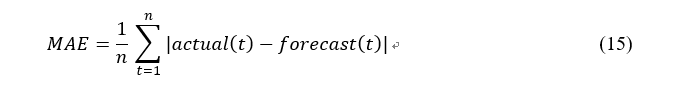import numpy as np

def mae_value(y_true, y_pred):
"""
参数:
y_true -- 测试集目标真实值
y_pred -- 测试集目标预测值

返回:
mae -- MAE 评价指标
"""

n = len(y_true)
mae = sum(np.abs(y_true - y_pred))/n
return mae


均方误差（MSE）：均方根误差（RMSE）

它表示误差的平方的期望值，它的计算公式如下 MASE is used to measure the deviation between the observed value and the true value. MASE is more sensitive to outliers. MASE is defined as follows：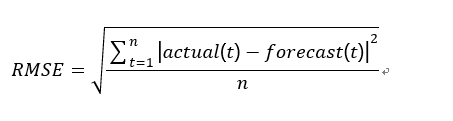import numpy as np

def mse_value(y_true, y_pred):
"""
参数:
y_true -- 测试集目标真实值
y_pred -- 测试集目标预测值

返回:
mse -- MSE 评价指标
"""

n = len(y_true)
mse = sum(np.square(y_true - y_pred))/n
return mse


平均绝对百分比误差 MAPE

MAPE  是 MAD 的变形，它是一个百分比值，因此比其他统计量更容易理解。例如，如果 MAPE 为 5，则表示预测结果较真实结果平均偏离 5 %。MAPE 的计算公式如下：MAPE is used to measure the relative errors between the average test value and the real value on the test set. MAPE is defined as: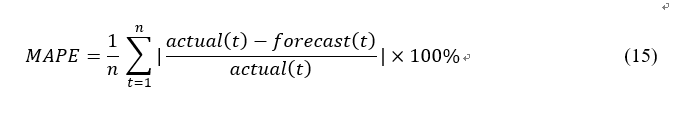MAPE 的值越小，说明预测模型拥有更好的精确度。使用 Python 实现 MSE 计算函数：

import numpy as np

def mape(y_true, y_pred):
"""
参数:
y_true -- 测试集目标真实值
y_pred -- 测试集目标预测值

返回:
mape -- MAPE 评价指标
"""

n = len(y_true)
mape = sum(np.abs((y_true - y_pred)/y_true))/n*100
return mape



展开全文•人工智能 机器学习
•人工智能 机器学习
• 前言：机器学习的主要任务是使用数据...本文汇总了最常用的几种评价指标，方便快速查询使用。（本文使用鸢尾花数据集和逻辑回归模型） 1.常用评估指标及损失函数： 1 import numpy as np 2 import pandas a...
•人工智能 机器学习
•人工智能 机器学习
• 模型的泛化性能进行评估，不仅需要有效可行的实验估计方法，还需要衡量模型泛化能力的评价标准 回归任务中最常用的性能度量是“均方误差”（MSE） 分类任务中的评价标准更为全面，下面着重介绍分类评价任务中的...机器学习 深度学习
• 数据包络分析(DEA)和回归分析(RA)两种方法都是处理多属性决策问题中常用的方法,前者的评价基准是基于“优秀者”,后者的评价基准是基于“平均像”.将这两种方法结合起来形成一个统一的评价方法,即DEARA方法,可以实现...
•人工智能 机器学习
• 这是《AI初识境》第10篇，这次我们说说深度学习模型常用的评价指标。所谓初识，就是对相关技术基本了解，掌握了基本的使用方法。 凡事用数据说话，一个深度学习模型在各类任务中的表现都需要定量的指标进行评估，...
• 回归模型评价指标中，常用的方法如下几种： MSE RMSE MAE R2 混淆矩阵(Confusion matrix) 在机器学习领域中，混淆矩阵（confusion matrix）是一种评价分类模型好坏的形象化展示工具。 混淆矩阵的定义 混淆矩阵...
• 在做回归建模相关任务时，最常用评价指标是MAE、MSE、RMSE中一个或多个，但如何根据自己具体任务场景(数据分布)选择更合适的模型评估指标指标呢？这里我们就要需要弄明白MAE与MSE之间到底什么不同。 1、什么...
•人工智能 机器学习
• 集成学习之优化基础...在回归中，我们最常用的评价指标为均方误差，即：，其中是样本 Xi应用建立的模型f预测的结果。如果我们所用的数据是训练集上的数据，那么这个 误差为训练均方误差，如果我们使用测试集的数据计人工智能 机器学习 python
•人工智能 机器学习
•人工智能 机器学习
• 模型评价指标2.1. 精度与召回率2.2 ROC曲线3. 模型选择 首先这篇文章是本人在学习机器学习时学习笔记，使用学习教材主要是雷明老师所编著《机器学习原理、算法与应用》一书。 1. 算法分类 机器学习算法按照...
•人工智能 机器学习
• 在工业界，逻辑回归是很常用的模型，一般大家在用逻辑回归做机器学习排序或者广告预估时常用AUC来判断排序的效果，逻辑回归是概率模型，除了排序的指标之外，有时会出现AUC比较好，但是概率拟合较差（很可能是收敛...
•人工智能 机器学习
• 1.什么是过拟合？什么是欠拟合？怎么样去诊断算法是否是过拟合或欠拟合？ 过拟合：训练误差很小，验证误差较大。高方差， 欠拟合：训练误差和验证误差都很大。...3.我们哪些指标评价一个模型的好坏？ 回归...
•人工智能 机器学习
•人工智能 机器学习
•人工智能 机器学习
• 评价指标包括客观度量和统计度 量,谱分辨率特性是客观度量中重要指标,而统计度量指标则 包括方差、均方误差等。 在频谱分析中主要包含两大类方法:古典谱估计和现代谱估 计。占典谱估计包括周期图估计法和相关法,...随机信号处理
• 集成学习-Task6度量模型性能的指标与贝叶斯优化调参概念介绍常用的评价指标贝叶斯超参数调优 度量模型性能的指标与贝叶斯优化调参 概念介绍 度量分类模型的指标和回归指标有很大的差异，首先是因为分类问题本身的...算法 机器学习
• 六、模型评估 模型评估是模型开发过程不可或缺一部分。它助于发现表达数据最佳模型和所选模型将来工作性能如何。...RMSE是一个衡量回归模型误差率的常用公式。 不过，它仅能比较误差是相同单位模型。 举